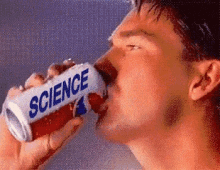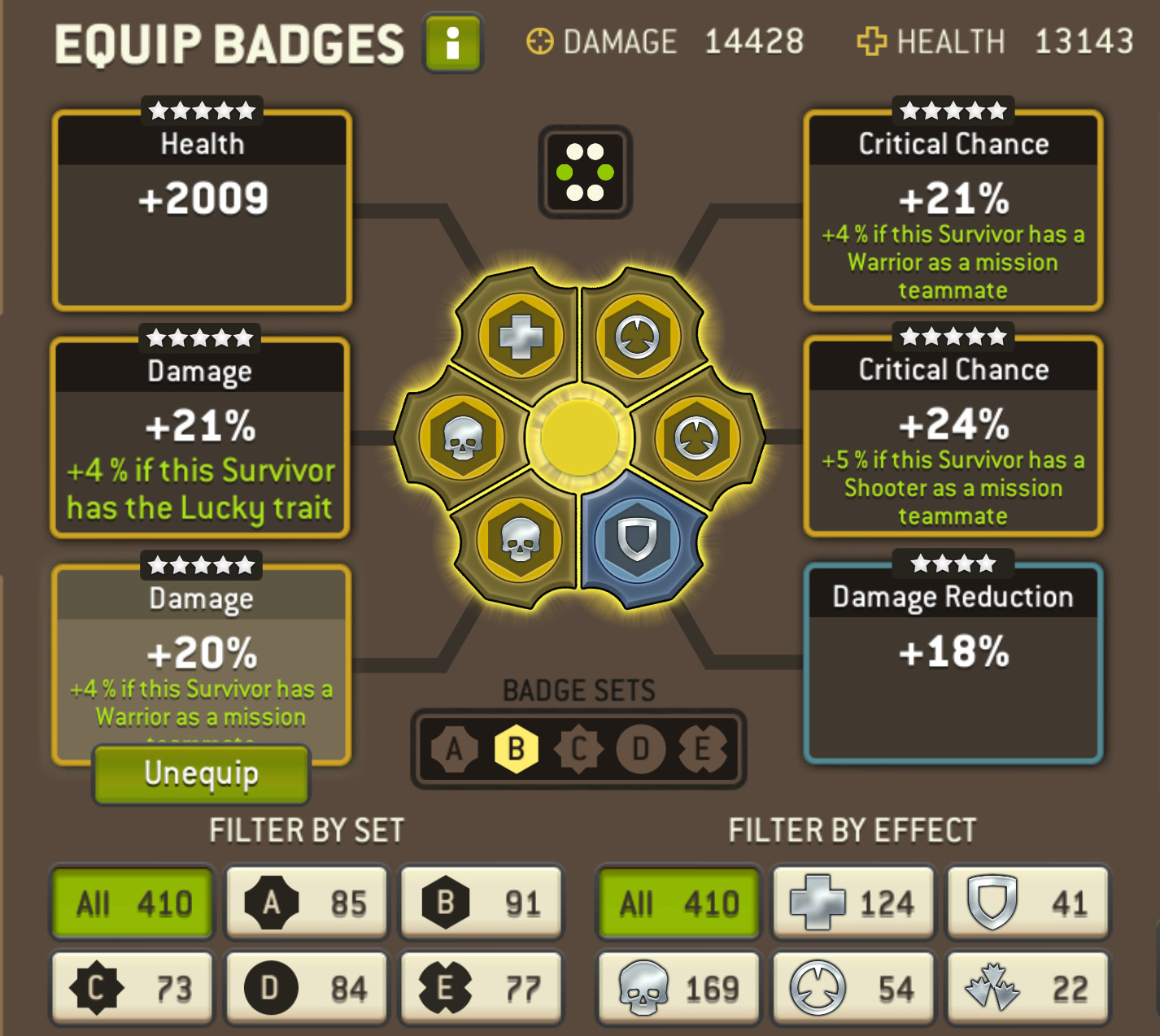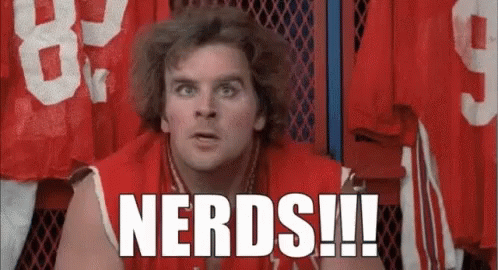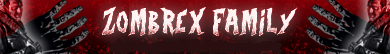# Survivor damage calculation - how to?

edited September 15
I've been trying to calculate the damage of a toon based on its current stats which are visible in the Training Grounds screens.

My formula does not seem to work, and I am too lazy and daft to figure it out. What is the correct formula please?

Damage = {BS*(1+SM)+BW+BF}*(1+LM)*(1+MM)*(1+BM)      (<== this formula is wrong!)
Edit:
Damage = (BS*(1+HM+SM)+BW+BF)*(1+LM+MM)*(1+BM) (<== this formula is correct! Thanks Burmeliinis!!)
Where
BS = Base Survivor Damage
HM = Hero modifier
SM = Star Modifier
BW = Base Weapon damage
MM = Marksman Modifier
LM = Lethal Modifier
All the modifiers are expressed as a multiplier, i.e. a 45% total badge effect is written as 0.45; 2 pink stars are written as 0.5.
The Star Modifier is 0.1 at 1 star, then increments to 0.4 at 5 stars in steps of 0.1. From 6 to 10 stars (corresponding to 1 to 5 pink stars) it increments in steps of 0.05. The Lethal, Marksman and Badge modifiers are said in their descriptions to act on the total damage.
I know my formula is wrong, because if I calculate a Base Survivor Damage for one configuration, and then on the same toon swap its weapon, or switch its badges, the outcome is wrong. Example:
=> Toon Alpha with two stars, Base Survivor Damage assumed to be 2539; Base weapon Damage = 4696; Lethal modifier = 0; Marksman Modifier = 0.17; Badges modifier = 0.45 => outcome = 14428 (matches displayed in-game value)
=> Toon Alpha with two stars, Base Survivor Damage assumed to be 2539; Base weapon Damage = 3950; Lethal modifier = 0.15; Marksman Modifier = 0.17; Badges modifier = 0.47 => outcome = 15345 (displayed in-game value = 15061)

Correct formula:
1) => Toon Alpha with two pink stars, Base Survivor Damage assumed to be 2273; Base weapon Damage = 4696; Lethal modifier = 0; Marksman Modifier = 0.17; Badges modifier = 0.48 => Damage = (2273*(1+0.1+0.5)+4696+0)*(1+0+0.17)*(1+0.48) = 14429 (matches displayed in-game value of 14428)
2) => Toon Alpha with two pink stars, Base Survivor Damage assumed to be 2273; Base weapon Damage = 3950; Lethal modifier = 0.15; Marksman Modifier = 0.17; Badges modifier = 0.504 => Damage = (2273*(1+0.1+0.5)+3950+0)*(1+0.15+0.17)*(1+0.504) = 15062 (matches displayed in-game value of 15061)
Caveat: Badge modifiers can be wrongly displayed due to rounding errors. For sure on Next Games' to-do-list...

• I wondered that too. My rick hits on crits between 180k and 230k (mostly) without pw. Why the difference? Its not the badges, i checked them.
• edited September 13
Profile picture damage is calculated as

(Base dmg + weapon dmg + flat dmg badges) * (Strong/Marksman + other dmg boosts + Hero LT dmg boosts)  * % dmg badges

The hard thing is the base damage. This is defined separately for each level and each class. So for Alpha (Hunter) at lvl 28, base damage is 2273. To that is then added a bonus for heroes, which for Alpha is 10%, and for stars 50% (40% for legendary and 5% for each pink star). So base dmg is 2273 * 1,6 = 3636 (at lvl 28).

Concerning @ladre5's question, there is a damage interval, which is usually 20% of weapon damage. So when you add all crit and charge boosts, the range between min and max starts to become considerable.
• I concur! 😌Leader of the original TWD guild (est. 10/2015).
We Are TWD 😎
FuriousMoldovan's NML Glossary
• Profile picture damage is calculated as

(Base dmg + weapon dmg + flat dmg badges) * (Strong/Marksman + other dmg boosts + Hero LT dmg boosts)  * % dmg badges

The hard thing is the base damage. This is defined separately for each level and each class. So for Alpha (Hunter) at lvl 28, base damage is 2273. To that is then added a bonus for heroes, which for Alpha is 10%, and for stars 50% (40% for legendary and 5% for each pink star). So base dmg is 2273 * 1,6 = 3636 (at lvl 28).

Concerning @ladre5's question, there is a damage interval, which is usually 20% of weapon damage. So when you add all crit and charge boosts, the range between min and max starts to become considerable.

Thanks Burmeliinis.
If I use the values I quoted for my lvl 28 Alpha with two pink stars I get
(Base dmg + weapon dmg + flat dmg badges) * (Strong/Marksman + other dmg boosts + Hero LT dmg boosts)  * % dmg badges
= ((2273*1.6)+4696+0)*(0.17+0+0)*0.45 = 637.5
If I enter the values in the formula I quoted, with the base damage multiplied with 1.6 rather than 1.5 to take alpha's 0.1 Hero multiplier into account I get
{BS*(1+SM)+BW+BF}*(1+LM)*(1+MM)*(1+BM)
= (2273*(1+0.6)+4696)*(1+0)*(1+0.17)*(1+0.45) = 14137
The displayed value is 14428.

• Yes so the 14137 is correctly calculated (my formula was simplified to show what to add, obviously badges etc are 1+the badge %). So there's 3 possible options why your Alpha's damage is higher.

2. Did you have a hero with a damage boost as leader?
3. Sometimes the badges are shown with an incorrect value.
• Two configurations:
1) Lvl 28 Alpha using a LVL31 weapon having 4696 base damage (no Lethal modifier)
Calculated damage = (2273*(1+0.6)+4696)*(1+0)*(1+0.17)*(1+0.45) = 14137
2) Lvl 28 Alpha using a LVL30 weapon having 3950 base damage (0.15 Lethal modifier)
Calculated damage = (2273*(1+0.6)+3950)*(1+0.15)*(1+0.17)*(1+0.45) = 14358• edited September 15

You have (18%+4%+18%) * 1.2 = 48.0%

Note that one of the badges probably is displayed as having a value of 17% but it actually is a 18% badge.

Also consider swapping out the Health and DR badge for another Dmg and CD badge

So then

1) Lvl 28 Alpha using a LVL31 weapon having 4696 base damage (no Lethal modifier)
Calculated damage = (2273*(1+0.6)+4696)*(1+0)*(1+0.17)*(1+0.48) = 14428

You have (18%+4%+18%) * 1.2 = 48.0%

Note that one of the badges probably is displayed as having a value of 17% but it actually is a 18% badge.

Also consider swapping out the Health and DR badge for another Dmg and CD badge

So then

1) Lvl 28 Alpha using a LVL31 weapon having 4696 base damage (no Lethal modifier)
Calculated damage = (2273*(1+0.6)+4696)*(1+0)*(1+0.17)*(1+0.48) = 14428

Smashing, configuration 1 now works very well! Thanks Burmeliinis!

The second configuration still does not play along, possibly by the lethal modifiers not being calculated correctly:
2) Calculated damage = (2273*(1+0.6)+3950)*(1+0.15)*(1+0.17)*(1+0.48) = 15108 (displayed 14821)
Further, if in configuration 1, I swap the +18-warrior badge for a +19% badge (total badge modifier = (18%+4%+19%)*1.2 = 49.2%, the calculation is off once more:
3) Calculated damage = (2273*(1+0.6)+4696)*(1+0)*(1+0.17)*(1+0.492) = 14546 (displayed is 14662
Any ideas on this plz?
•Lol
I think there's two types of players, those that care about this sort of stuff and those who couldn't care less. I am definitely the latter!

If damage was fixed I might pay attention as I might be able to make a choice in a mission to target a certain walker knowing how much damage I'm going to do. Since there is such a huge range with base damage affecting your range with a charge it is absolutely useless to know these sort of things IMO• WellyLuga said:
I think there's two types of players, those that care about this sort of stuff and those who couldn't care less. I am definitely the latter!
Good for you then. Thank you for expressing yourself.
• 3) (2273*(1+0.6)+4696)*(1+0)*(1+0.17)*(1+0.492) = 14546 (displayed is 14662)• For case 2

2) Calculated damage = (2273*(1+0.6)+3950)*(1+0.15+0.17)*(1+0.48) = 14820

For case 3, check carefully what the badge's unboosted value is, maybe it's not actually 19% boosted?

• Case 2.. sorted! Thanks ever so much!!
Case 3: pic below is the badge. For the formula to work for case 3 the badge ought to have a 20% modifier, rather than the displayed 19%.• That’s it then. NG is known for their wacky roundings so it seems you got two badges that are displayed with a 1% too low value…
• That’s it then. NG is known for their wacky roundings so it seems you got two badges that are displayed with a 1% too low value…
Awesome. So I guess the only way to find the true Survivor Base damage is to strip them of all their badges and then apply the formula, with the total damage given and solving for the Survivor Base damage.
With that given, the badge display errors could technically be worked out. Pain in the neck though.
•  Good for you that is all resolved already 😉 you can find all base damages here: https://docs.google.com/spreadsheets/d/1FIr5GaV3V1mLkqA6zDjucJjzJKKPupeInfuGEklpreE/edit
•  Good for you that is all resolved already 😉 you can find all base damages here: https://docs.google.com/spreadsheets/d/1FIr5GaV3V1mLkqA6zDjucJjzJKKPupeInfuGEklpreE/edit

Yessss.... that helps too! Much appreciated. Not too sure what I'll do now during the next badge event• One more thing, consider joining magic’s discord if these kinds of things interest you: https://discord.gg/m5DaGZ22
• WellyLuga said:Lol
I think there's two types of players, those that care about this sort of stuff and those who couldn't care less. I am definitely the latter!

If damage was fixed I might pay attention as I might be able to make a choice in a mission to target a certain walker knowing how much damage I'm going to do. Since there is such a huge range with base damage affecting your range with a charge it is absolutely useless to know these sort of things IMOYea I’m just having some fun. I do like the statistical aspect but get frustrated by it because it’s like flipping a coin at times.
Revenge of the Nerds movie is a classic lol
My inner Jock had to post this Meme haha
•Dude... where's my car?#Zombrex (Neo / Horizon / (OG) / Genesis / Prime / Elite)

Are you Lost? Alone? Looking for a killer team to have your back?
Join ZOMBREX! We have a tiered guild structure so players of every level and ambition can find a home they fit in.
Remember, search ZOMBREX FAMILY.
Our page :